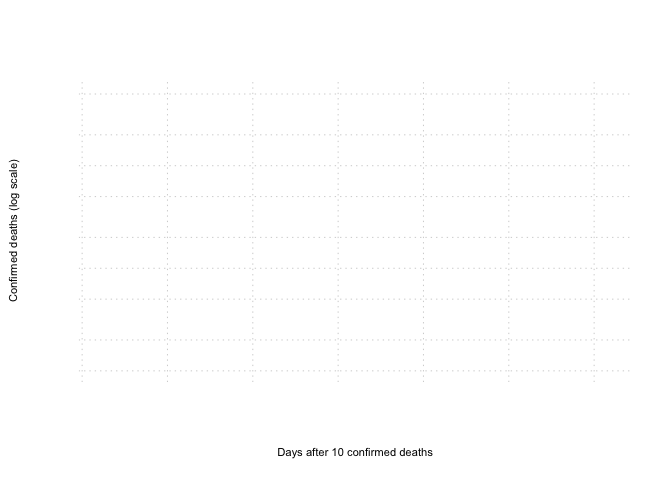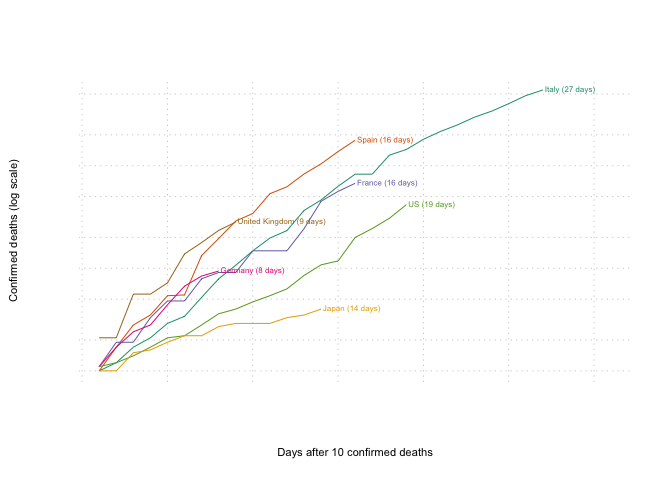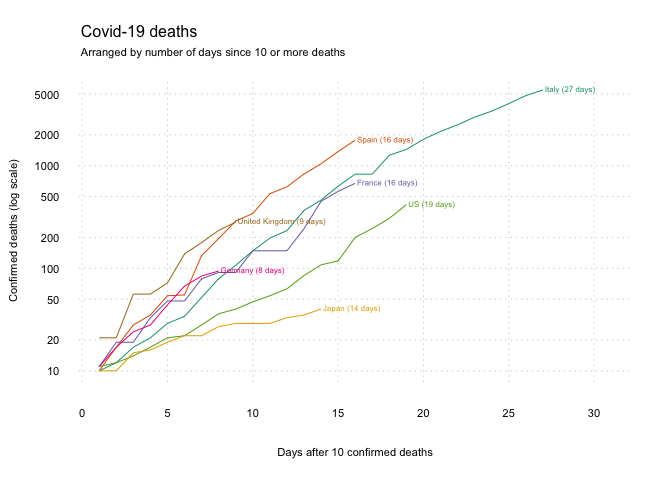(Note that if you don’t need any help with R you can find the complete script here)

Keeping up with what is going on with Covid-19 is challenging. Trends in the number of cases can be difficult to interpret as testing varies between countries and over time. So while in South Korea it is possible to get tested if you have even relatively mild symptoms, or where exposed to someone who is ill, in the UK you won’t be tested unless you need hospitalisation with severe symptoms. It is possible that the UK will change its approach to testing, to include milder cases, in which case the number of confirmed cases would rise even if the epidemic hadn’t changed. Likewise, in Korea, if they decided to cut back on testing to only severe cases, the number of confirmed cases would fall without any change in the spread of the disease. Therefore, the number of confirmed cases is not in ideal way to compare the growth of cases in different countries.

Because people with severe symptoms are being tested in high-income settings, the number of Covid-19 deaths is a more consistent indicator of the progression of the pandemic. These figures should also be interpreted with caution since the absolute number of deaths does not account for differences in the population sizes of different countries. However, one aspect of the trend does not depend on the size of the population (at the start of the epidemic) is the growth rate: how much the number of deaths is changing over time. Ordinarily we would think of the rate of change as the slope of a line showing how the number of deaths is changing with time. However, because each case with the virus infects around two other people, the growth is exponential, and the slope of the line showing the absolute number of deaths itself increases with time. To account for the fact that it is the relative change in the number of deaths that matters, we can take the logarithm of the number of deaths, which undoes the exponent so that the trend (in log deaths) is linear.

Graphs of the log number of deaths by country can be found in the media – although many of the better onese are behind paywalls. It is interesting, and perhaps useful, to be able to produce these graphs oneself. Fortunately, Johns Hopkins University in Baltimore, US, publishes an updated table of cumulative deaths each day, for each country in the world. We can use these data to produce a graph of the log deaths in whatever countries we choose.

While it is possible to download the data and create graphs with Excel or in Google Sheets, a very popular software for the analysis of data is the open-source statistics platform R. R takes a little bit of learning, but is widely favoured over other (expensive) statistics platforms.

In the following sections, I will explain how you can get started in R and, for your first project, produce a graph of Covid-19 deaths. Please note: this is not a complete intro to R, and you may need to ask Google how to do the things that you want to do.

## Getting started

To get started you will need R installed on your computer. The easiest way to do this is to download and install RStudio. RStudio comes with R, but it is also an easy-to-use interface for using R, with tonnes of helpful features (and it’s free). So click on this link and install RStudio on your computer.

At this point you should have RStudio installed and open on your computer. All of the remaining steps are written in R. They are coded instructions to R about where to get data and what to do with it. We can save a series of instructions in a .R script, like a recipe for what we want R to do. You can open a new .R script by going to File > New File > R Script. All of the coded instructions are included in this post, below. You can either write these out into your .R script, or you can copy-paste them over. Alternatively, you can copy-paste all of the code in one go by going here.

R makes it really easy to load data. The read.csv() command can find a .csv file on your computer or, helpfully, at a URL online. When read.csv() loads in the data at the URL below, we assign this data to the name data_raw using the <- symbol.

data_raw <- read.csv("https://raw.githubusercontent.com/CSSEGISandData/COVID-19/master/csse_covid_19_data/csse_covid_19_time_series/time_series_19-covid-Deaths.csv")

## Data management

Before we can produce our graphs, we need to do a little data management. These steps will select the bits of the dataset that we actually need, and do some re-organising. We aren’t changing any of the underlying values in the data.

### Selecting the countries

Step one is to determine which countries we want to look at. We make a list of the names of the countries, using c(), and assign this to the object countries. We then select those counties from the raw data (data_raw). This is done by asking R to check if each row of the ‘Country.Region’ column is ‘in’ (%in%) the list of countries that we just made (countries). We can assign this reduced dataset with only the countries we want to the object called data. And to simplify things, we can remove columns that we won’t be using.

# Names of selected countries
countries <- c("Italy","Spain","France","Germany","US","Japan","United Kingdom")

# Keep data for selected countries
data <- data_raw[data_raw$Country.Region %in% countries, ] # Keep only country (column 2) and the columns # with the cases per day (columns 5 onward) data <- data[, c(2, c(5:ncol(data)))] ### Re-organising the data If you type View(data) into the Console in RStudio, a new tab will appear and you will be able to see what data looks like. You will see that each row is a country, and most of the columns are dates. (Note that for the US each state is reported separately but they will all be combined later.) Rather than having all of the deaths in different columns, it is easier to use the data to make a graph if we have the number of deaths in one column and a new column with the date. Each country will have multiple rows associated with it, one for each date. We do this re-organisation with reshape(). It is not 100% necessary to understand how reshape() works, but after running it you can use View(data) again to see how the data has changed. # Change the data from 'wide' to 'long' data <- reshape(data = data, direction = 'long', varying = c(2:ncol(data)), sep='', new.row.names=NULL) ### Dates and grouping The final step before we can actually plot the data is to tell R that the time variable is supposed to be a date, and to ensure that each country only has one entry per day (looking at you, USA). The latter is achieved with aggregate(). Finally, since we are only interested in the state of the epidemic once local transmission started, we set an arbitrary minimum number of deaths. Doing so means that we are plotting the trend in deaths after at least ten deaths were recorded in the country. # Identify the dates as dates in the data data$time <- as.Date(data$time, "%m.%d.%y") # Group the data by country and time, with the sum of cases data <- aggregate(data$X,
list(data$Country.Region, data$time),
'sum')

# Only keep days with 5 or more deaths

plot(plot_data$x, type = 'n', log = 'y', # y-axis is on the log scale bty = 'n', # no border around the plot xlim = c(1,(nrow(plot_data)+4)), axes = FALSE, bg='gray80', xlab='Days after 10 confirmed deaths', ylab='Confirmed deaths (log scale)', cex.lab=.7) # Add gridlines grid(nx = NULL, ny = NULL, col = "lightgray", lty = "dotted", lwd = par("lwd"), equilogs = F)### Adding the trend lines Finally, after all that, we are ready to add the trend lines. To do this, we tell R to select the data from data for each country in countries and plot the lines. The for(){} code tells R to do the code inside of the curly brackets once for each country in countries. The colour of the line is taken from the cols that we produced earlier. After plotting the line, we also add a label to the final point using text() with the name of the country and the number of days since five or more cases were recorded there. # Add each of the other countries for (country in countries){ plot_data <- data[data$Group.1 == country,]
# Plot the lines
lines(c(1:nrow(plot_data)), plot_data$x, col=cols[which(countries==country)]) # Add a label text(x=nrow(plot_data), y=max(plot_data$x),
label=paste0(country,' (',nrow(plot_data),' days)'),
pos=4, offset=.1, cex=.5,
col=cols[which(countries==country)])
}### Final touches

To finish up, we can add the axes and titles. And we’re done.

# Add the axes
axis(1, lwd=0, cex.axis=.7)
axis(2, lwd=0, las=1, cex.axis=.7)

mtext(side=3, line=1, at=-0.07, adj=0, cex=0.7, mysubtitle)